Quadratic Inequalities Terms of Use    Contact Person: Donna RobertsDirections: Grab paper and pencil. Answer the following questions pertaining to quadratic inequalities. Check your answers when you are finished with each question.

1.
Which choice is the solution to the inequality:
x2 + 2x - 15 < 0 ?Choose:
 -3 < x < 5 -5 < x < 3 x < -5 or x > 3 x < -3 or x > 5

2.
Which choice is the solution to the inequality:
x2 - 4x - 5 < 0 ?Choose:
 {x | -1 < x < 5} {x | x < -1} {x | -5 < x < 1} {x | x < -1 or x > 5}

3.
Which inequality's solution is shown by this graph?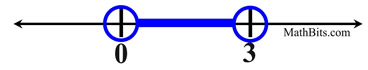Choose:
 x2 + 3x > 0 x2 - 3x > 0 x2 + 3x < 0 x2 - 3x < 0

4.
Which graph represents the inequality:   y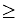x2 - x - 6 ?
Choose: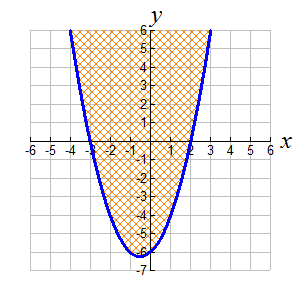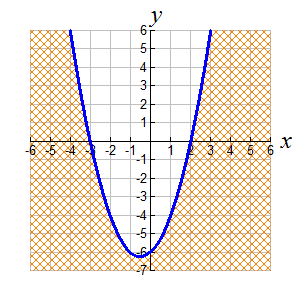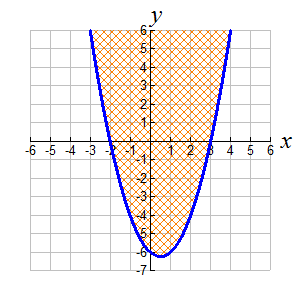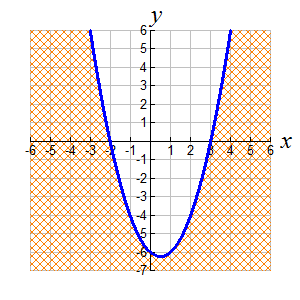5. a.) Graph the inequality y < x2 - 7x + 10 ? b.) Is the point (-4,-8) in the solution set? c.) Is the boundary line drawn solid or dashed? Explain.6. A tennis ball is hit into the air. The height of the ball, h(t), in feet, at time t, in seconds, is modeled by the equation h(t) = -16t2 + 64t + 4. Determine the interval of time when the height of the ball is greater than or equal to 52 feet?7.
Which inequality is graphed at the right?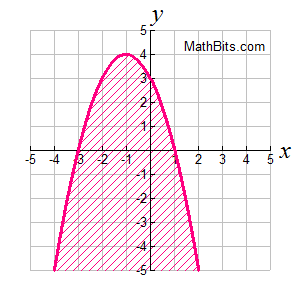Choose:
 y < -x2 - 2x + 3 y > x2 - 2x - 3 y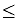-x2 - 2x + 3 yx2 - 2x - 3